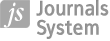RESEARCH PAPER
Optimal Investment Rates in an N-Capital Growth Model

More details
Hide details

Publication date: 2007-09-30

GNPJE 2007;218(9):1–18

KEYWORDS
ABSTRACT
The paper aims to determine an optimal structure of investment rates under an N-capital growth model. This model is a combination and expansion of models developed by Solow ; Mankiw, Romer, Weil ; Nonneman, Vanhoudt ; and Tokarski [1996; 2000; 2003; 2005], with elements of optimal control models by Ramsey , Lucas  and Romer [1986, 1990]. The economic growth model described in the paper is based on the following assumptions: - The stream of products generated in the economy is influenced by a finite N amount of capital, labor and technology resources; it is also assumed that labor and technology resources grow according to certain exogenous growth rates; - The increase of each of the analyzed capital stocks is the difference between investment in this capital and its depreciation; these assumptions refer to the neoclassical growth models developed by Solow, Mankiw-Romer-Weil, and Nonneman-Vanhoudt; - A typical rationally behaving consumer (much as in the case of endogenous growth models developed by Lucas and Romer) seeks a long-term investment rate structure that will maximize the usefulness of consumption in an infinite period of time; - Additionally, an assumption is made that the macroeconomic production function in the described growth model does not have to be characterized by a constant scale effect (as earlier noted by Tokarski [1996, 2003 or 2005]). The model described by the author is solved using Pontryagin’s maximum principle.
 eISSN: 2300-5238